# Musings on Large Numbers

Anyone who has taken an introductory course in probability, or even SPC, has heard of the law of large numbers. It’s a powerful result from probability theory, and, perhaps, the most widely used. Wikipedia starts the article on this topic with a statement that is free of any caveat or restrictions:

In probability theory, the law of large numbers is a theorem that describes the result of performing the same experiment a large number of times. According to the law, the average of the results obtained from a large number of trials should be close to the expected value and tends to become closer to the expected value as more trials are performed.

This is how the literature describes it and most professionals understand it. Buried in the fine print within the Wikipedia article, however, you find conditions for this law to apply. First, we discuss the differences between sample averages and expected values, both of which we often call “mean.” Then we consider applications of the law of large numbers in cases ranging from SPC to statistical physics. Finally, we zoom in on a simple case, the Cauchy distribution. It easily emerges from experimental data, and the Law of Large Numbers does not apply to it.

## Averages Versus Expected Values

Hans Rosling was most eloquent on the value and limitations of averages.  He did not, however, relate them to expected values:

Averages, or arithmetic means, are functions of your data that you can calculate with built-in functions in electronic spreadsheets. Expected values, on the other hand, are attributes of probability models, not data. Calling them means makes them easy to confuse with data averages. And they don’t exist for every model.

On Wikipedia, you find formulas for the expected values of many distributions under “mean” in the right sidebar. In the following examples of these sidebars. note that the mean for the Cauchy distribution is “undefined”: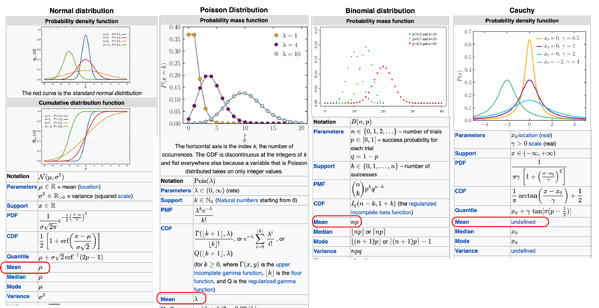From the literature on statistical quality, you would never guess that there are probability distributions with no expected value. Yet the Cauchy distribution is not exactly exotic, and has been studied for 200 years. Its simplest form, the standard Cauchy distribution, is simply the ratio of two centered, independent Gaussians with unit variance. You don’t usually apply Student’s t-test to a sample of only two points but, if you do, you find out that Student’s t-distribution with a single degree of freedom is identical to the standard Cauchy distribution.

A Cauchy variable has no expected value. Therefore the law of large numbers does not apply to it, and neither does the Central Limit Theorem. Sample averages do not converge, and sums of independent Cauchy variables do not approximate Gaussians.

### Expected Values

Having established that many but not all probability distributions have an expected value, let’s briefly review what it is and how you calculate it.

#### A simple bet

If you make the same bet at roulette n times, and your winnings are  \left ( w_{1},\dots, w_{n} \right ), you can take their average, or arithmetic mean

\bar{w} = \frac{w_{1}+\dots + w_{n}}{n}

If you win w with probability P\left (win\right ) and you lose l with probability P\left (loss \right ) = 1- P\left ( win  \right ), then your winnings on one bet is a random variable W, and its expected value is

E\left ( W \right )= w\times P\left (win\right ) - l\times P\left (loss \right )

The law of large numbers then says that, as you repeat your bet more and more, the average winnings —  a function of your data — converge to their expected value — a mathematical attribute of their distribution.

#### The general case

If you have more than two possible outcomes, it becomes a sum of all these outcomes. If you have a random variable X taking a continuum of values with the probability distribution function f it becomes

E\left ( X\right )= \int_{-\infty}^{+\infty}xf\left ( x \right )dx

if this integral converges. If it doesn’t, the variable does not have an expected value, and the law of large numbers does not apply. This extends to multidimensional variables, random processes, and random functions.

### Use of the law of large numbers

Where it applies, the law of large numbers lets you use averages as estimates for unknown expected values. The key question is how large a sample needs to be. We also need to keep in mind how sensitive averages are to extreme values. For variables that have standard deviations, it is commonly used to characterize the precision of the estimation as a function of sample size. If \sigma is the standard deviation of individual values, then the standard deviation of the average of  n independent values is \frac{\sigma}{\sqrt{n}}.  Different applications work with samples of different sizes.

#### SPC

In SPC, as set forth 100 years ago, to set the center line on the \bar{X}-chart of a critical dimension, you apply this law to a sample of measurements that you deem representative of the process, sometimes as small as 30 points. It wasn’t large but, in the 1920s, you couldn’t practically do much more. Manually collecting and analyzing this many points on hundreds or thousands of critical dimensions in a plant was a large task. With 30 points, the standard deviation of the average is only \sqrt{30} = 5.48 times smaller than for individual values; if you automatically captured this dimension on each of the 1,000 units made in a day, it would be  \sqrt{1000} = 31.62 times smaller.

#### Political Polling

Political pollsters ask presumably representative samples of ~2,000 likely voters whether they would vote for Candidate X  if the election were held that day. Then the results are used to predict how 200 million voters would vote. It differs from a critical dimension in that the random variable you average is the indicator of a voter’s choice. It’s worth 1 if it is Candidate X and 0 otherwise. The expected value of this indicator is the probability that a voter will choose Candidate X. Over 2,000 voters, the standard deviation of the average is 44.7 times smaller than for an individual voter. The issues of using such an estimate on the much larger population of all voters is discussed for a different application in a previous post on acceptance sampling.

#### Epidemiology

Epidemiologists working on the COVID-19 pandemic had data on hundreds of millions of people. Epidemiological models like SIR treat the numbers of Susceptible, Infected, and Recovered people as real numbers even though they are integers, and express their relationships over time through differential equations. That’s because these relationships are not between the actual numbers but their expected values. With numbers this large, the distinction between the proportion of people in one group and its expected value is negligible. This is the public health perspective. A susceptible individual, on the other hand, translates it to the probability of catching the disease.

#### Statistical Physics

Statistical physicists of the early 20th century did the opposite of statisticians, who infer population characteristics from data on individuals. The physicists instead inferred characteristics of individual molecules, atoms, or particles from observations on aggregates, like temperature, pressure, or radiation. “Large,” or even “big data” does not begin to describe populations like 6.02\times 10^{23} atoms in 1 gr of hydrogen, not individually observable. There is no point here in discussing convergence, as averages and expected values are indistinguishable.

#### Simulations

In the dawn of the computer age, the late 1940s, Stan Ulam thought of using this new tool to simulate random variables and use the law of large numbers to approximate parameters of nuclear reactions that were otherwise too difficult to calculate. This was key to the development of the H-bomb. The principle is simple and can be illustrated by estimating \pi from dart throws.

Assume the target is square, and that your aim is so bad that each dart has a uniform probability of landing anywhere on the square. If you have a circle inscribed inside the square, the ratio of its area to that of the square is \frac{\pi}{4}. It is also the probability that a dart will land inside the circle and the expected value of the ratio \frac{c}{n} of the number cof darts inside the circle to the total number n of darts thrown. By the law of large numbers, \pi = 4\times\lim_{n \to \infty} \left( \frac{c}{n} \right)

After 100 throws, the target looks like this: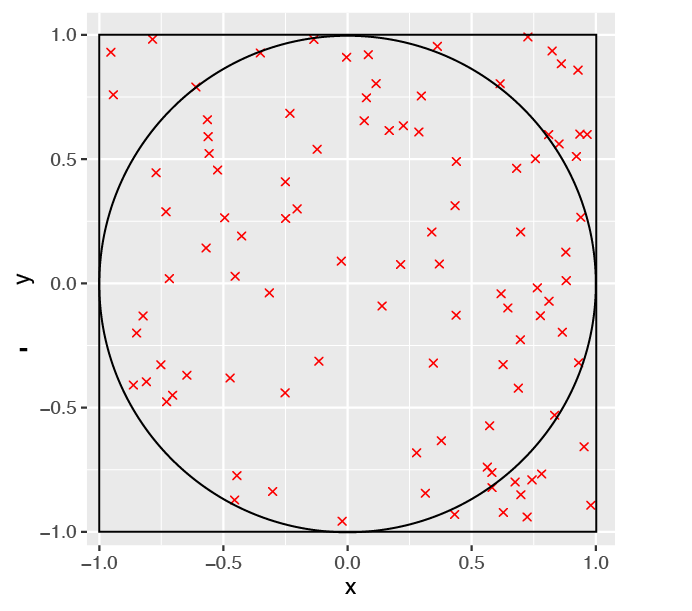With just 100 dart throws, the estimate of \pi is 3.04. With 100,000 throws, you get 3.14; with 100,000,000 throws, 3.1416. While 100 million dart throws might take a lifetime for a human, my simulator ran them in less than a minute.

By comparison, the approximation of \pi built into the R language is 3.141593, and the one used by NASA to calculate interplanetary flights is 3.141592653589793.

Ulam called his approach the Monte Carlo method. The term is still in use but no longer has a precise meaning.

### Cauchy distribution simulation

In the discussion of Averages of Manufacturing Data, I introduced the example of a rectangular plate with width and length as critical dimensions, On each unit, you measure the differences between their actual lengths and widths and the specs, as in the following picture:Assume then that, rather than the discrepancies in length and width, you are interested in the slope \frac{\Delta W}{\Delta L} and calculate its average over an increasing number of plates. If  \Delta W and \Delta L are both centered Gaussians with unit variance, their ratio is a standard Cauchy variable, and easily simulated. The following figure plots the evolution of the average m_n of the n first values in one sample of 10,000 points: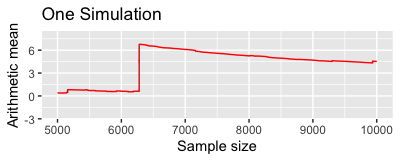It appears to converge to a value of around 4.5, leaving you wondering why. Running multiple simulations makes it clear that it actually does not converge as the law of large numbers had led you to expect:If the law of large numbers applied, these lines would all converge to the same limit, which they don’t. The data are independent instances of the standard Cauchy distribution by construction. If this distribution had an expected value, the law of large numbers would apply. The  m_n curves all appear to flatten as  n rises but it is, in fact, because they are, by construction increasingly autocorrelated:

m_{n+1} = \frac{n\times m_n + s_{n+1}}{n+1}

where   s_{n+1} is the next value. In spite of this, the denominator is occasionally small enough to cause a sudden rise, as seen in the red line after >6,000 points.

This chart, however, is not proof that the ratio has no expected value. Maybe the averages just haven’t converged yet and would if we simulated 100,000 or 1,000,000 points.

This kind of simulation can now be run on ordinary business laptops and has become ubiquitous. Changing parameters on a draft tax return to see the impact on amounts due is often called a “simulation.” What we are talking about here is different, as it involves generating pseudo-random numbers. It is now quick and easy for a broad range of distributions.

Many tools of data science now rely on simulation, including MCMC, bootstrapping, its special case bagging,  and a special case of bagging called random forest.

## The Math of the Cauchy distribution

To know for sure, we need to work out the math of the Cauchy distribution. Fortunately, it is relatively simple.

### The probability and cumulative distribution functions

Let’s consider it geometrically. We are looking for the distribution of S=\frac{Y}{X} when  X and  Y are independent, centered Gaussian variables with unit standard deviation. The joint distribution of \left ( X,Y \right ) is shown by the circular cloud in the figure, and the value of  S is constant along the line through the origin that make an angle \theta with the  x-axis such that  tan \left (\theta \right ) = \frac{y}{x}.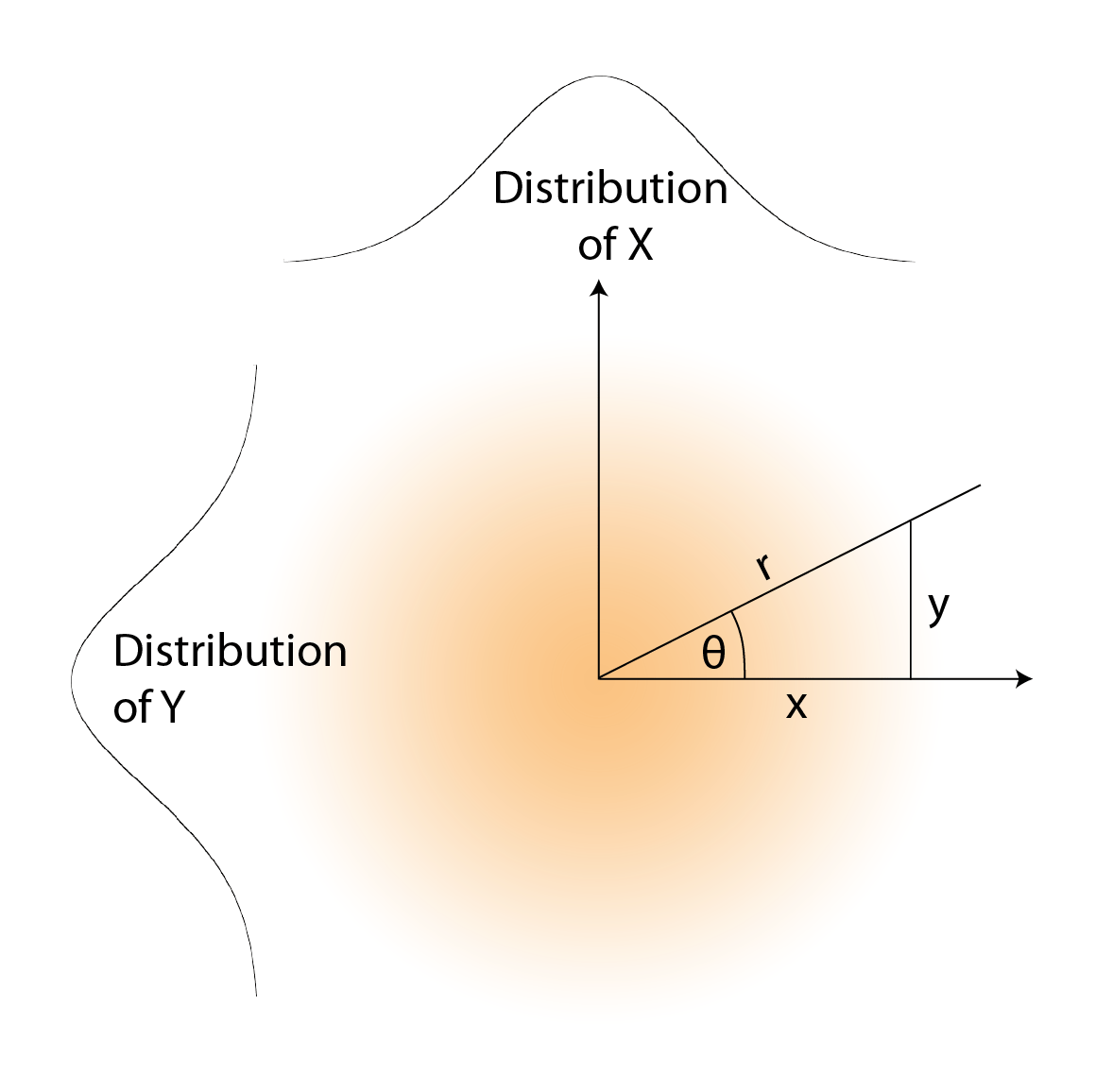To understand why the Cauchy distribution has no expected value, you need to recall a few results from trigonometry. You may not have used them for decades but they are, in fact, in deep storage in your memory:Specifically, for an angle \theta , if  s = tan\theta , then

\frac{\mathrm{d s} }{\mathrm{d} \theta} = \frac{1}{cos^2\theta} = 1 + tan^2\theta

and, conversely,  \theta = arctan\left ( s\right ) and

\frac{\mathrm{d \theta} }{\mathrm{d} s} = \frac{1}{1+s^2}

You can deduce the distribution of the ratio S = \frac{Y}{X} of two independent Gaussian variables X  and Y with 0 mean and unit variance from their joint distribution, by two changes of variables. In cartesian and polar coordinates, the integrand in probability calculations is of the form:

C\times e^{-\frac{x^2 + y^2}{2}}dxdy = C\times e^{-\frac{r^2}{2}}rdrd\theta

where r = \sqrt{x^2+y^2} and  \theta = arctan\left (\frac{y}{x} \right )

If we then replace  \theta with  s = \frac{y}{x} =  tan(\theta) then, since \frac{d\theta}{ds}= \frac{d}{ds}arctan\left ( s \right ) = \frac{1}{1+s^2}   we have:

C\times e^{-\frac{r^2}{2}}rdrd\theta = C\times re^{-\frac{r^2}{2}}\frac{1}{1+s^2}drds

The variables  and  separate and, by integrating over  for  r = 0  to   \infty , we are left with a probability distribution function for   S  of the form

f\left ( s \right ) = C'\times \frac{1}{1 + s^2}

and a cumulative distribution function of the form:

F\left ( s \right ) = C'\times arctan(s) +D

By normalizing, we get

f\left ( s \right ) = \frac{1}{\pi}\times \frac{1}{1 + s^2}

and

F\left ( s \right ) = \frac{1}{\pi}\times arctan(s) +\frac{1}{2}

### Weird properties of the Cauchy distribution

A careless analyst could easily mistake the treacherously bell-shaped Cauchy distribution for a Gaussian. It’s just pointier in the middle and has longer tails on both sides, and it has different and surprising properties.The first surprise is that is has no expected value. It explains why the law of large numbers doesn’t apply to the Cauchy distribution, If   S follows the standard Cauchy distribution, then

E\left ( S \right )=\frac{1}{\pi}\int_{-\infty}^{+\infty}\frac{s}{1+s^2}ds = \frac{1}{2\pi}log\left ( 1+s^2 \right )\bigg\rvert_{-\infty}^{+\infty} = \infty - \infty

which is undefined.

The other property, which is slightly more difficult to prove, is that the average of  n independent standard Cauchy variables follows the same distribution as the individual values. It’s still a standard Cauchy variable!

### Alternate use of the law of large numbers

As Geoffrey Johnson pointed out, there is another application of the law of large numbers that works with the Cauchy distribution. Instead of taking averages of the values, you count the number u   of data points out of the first n that are between values a and  b . Then the ratio  \frac{u}{n} does converge towards the probability that the variable falls between  a and  b .

### Detecting Cauchy

If all you have is a data set, how can you tell it’s a sample of Cauchy variables? A histogram may let you see the difference with a Gaussian, but a KDE may show the tell-tale pointy center and long tails more eloquently. Here is an example of both techniques applied to the same sample of 1,000 points, 20 of which fell outside the [-25, +25] range.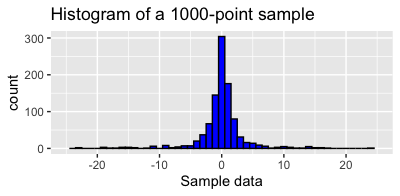Then you need to check the backstory of the data.

## Conclusions

Learn from your data, but check their backstory to make sure appearances don’t deceive you.